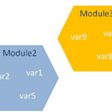# [Python Basic] Working With Lists — Part 2

We already know what the Python list is and how to manipulate one with some functions and even list comprehensions. Do we?

For many of you, it is true. But there is still a growing number of new generations who want to participate in this fascinating new world of machine learning, artificial intelligence, data science or whatever. We should provide them some materials to read, think and learn.

This post is an attempt to prepare teaching materials to my students whose major is not computer science, but who are willing to learn this new fascinating world.

# Making Numerical Lists

There are many reasons to store a set of numbers in computer programmings. Lists are ideal for storing sets of numbers and Python gives us tools to work with lists of numbers.

Using the `range()` Function

Python’s `range()` function makes it easy for us to create a series of numbers. With the help of `for` statement, we can see values in the `range()` function.

`for value in range(1,5):    print(value)`

One of the must-remember things in Python is the indexing scheme of its own. It might help us to remember that `a <= x < b` in `range(a, b)` . That is, the left-hand side is included, while the right-hand side is excluded. So the output will be 1, 2, 3 and 4, excluding 5.

Using the `range()` to Make a List of Numbers

If we want to make a list of numbers, we can do it using the `list()` function as we did in the previous posting.

`my_numbers = list(range(1,5))print(my_numbers)`

The protocol of `range()` function is `range(a, b, c)` , where `a` is the starting number, `b` is the ending number `+1` (remember the exclusion) and `c` is the increment. Some examples.

`range(1,5)                 # 1, 2, 3, 4range(2,7,1)               # 2, 3, 4, 5, 6range(3,10,2)              # 3, 5, 7, 9`

Here is a question where we can test our logical thinking. What is `range(3)` ? We are given the `range()` function with just one parameter? Is it a starting number, an ending number `+1` or an increment? If it were a starting number, we will get an infinite sequence starting from 3, which is impossible. If it were an increment, then we still do not have the ending number, we can guess the starting number might be 0 though. So the final candidate is that 3 is the ending number `+1` . Yes, `range(3)` is 0, 1 and 2.

Using `enumerate()` Function

It is useful to obtain an indexed list. It returns a pair of `(index, value)` in a loop. For example, modifying the previous post’s code with `enumerate()` function produces the index of the list as well as the value of the list.

`my_string = "Hello, Python!"my_list = list(my_string)for i, item in enumerate(my_list):    print(i, item)`

Remember that `enumerate()` always returns a pair so we need two variables (`i` and `item` in the code) to store the pair. Also, don’t forget the Python index starts at 0.

# List Comprehensions

There are many reasons why people love Python, and list comprehensions must be one of them. It makes our code shorter while maintaining the readability once we master it.

Let us consider the following example of finding squared numbers from 1 to 10.

`squares = []                   #1for value in range(1,11):      #2    squares.append(value**2)   #3print(squares)                 #4`

We make an empty list named squares at #1. Then using the for `loop` and the `range()` function at #2, we make the list containing squared values from 1 to 10 at #3. Finally, print the result.

Just for a quick reminder, `value**2` is a square operation and `squares.append()` is used for adding new values to the existing list.

With list comprehensions, we can make one line code for #1, #2 and #3.

`squares = [value**2 for value in range(1,11)]print(squares)`

Isn’t it nice to have a shorter code while keeping the readability? We don’t have to initialize the list and don’t have to use the append method. Compare the list comprehension with the three lines at #1, #2 and #3.

--

--

--

## More from A Ydobon

Ydobon is nobody.

Love podcasts or audiobooks? Learn on the go with our new app.

## Memento Design Pattern ✨😎## Alvin’s Dew Drop Daily — Issue #174## Mito: One of the Coolest Python Libraries You Have Ever Seen## An Unreal Experience — Alpha Build## A Few Java Brain-Teasers## Best Tech Stack for Startups?## A Ydobon

Ydobon is nobody.

## Python in a few wards 💻## Taking a snapshot of the browser in case of error using Selenium and pytest## Functions ()